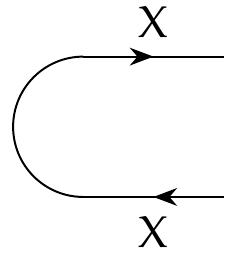Michael Hong wrote:

> So for this cap, we have \$$\cap_X (x,x') = \hom(x',x) = \cup_X (x,x')\$$ ?

Right.

I find the arbitrary conventions in this course endlessly confusing, first because there are \$$2^n\$$ options some fairly large value of \$$n\$$ (like 10), second because there aren't completely standard choices in every case, and third because using Fong and Spivak's textbook I don't feel completely free to make the choices _I_ think are best!

For example: I don't like string diagrams going left to right; I like them going top to bottom. So when I say the 'cap'

$\cap_X \colon \textbf{1} \nrightarrow X \times X^{\text{op}}$

is drawn like this:I'm already annoyed that the cap isn't being drawn as an actual cap \$$\cap\$$. And I'm also annoyed by the fact that this convention doesn't turn into my usual convention just by rotating the picture 90 degrees... I could explain that, but never mind.

But I can live with all this.

On a separate note, I really want the cap to go like this:

$\cap_X \colon \textbf{1} \nrightarrow X \times X^{\text{op}} \qquad (GOOD)$

and not this:

$\cap_X \colon \textbf{1} \nrightarrow X^{\text{op}} \times X \qquad (EVIL)$

because in the category of finite-dimensional real vector spaces we have a similar such thing for any object \$$V\$$:

$\cap_X \colon \mathbb{R} \to V \otimes V^\ast$

where \$$V \otimes V^*\$$ is the space of linear transformations of \$$V\$$ (or crudely speaking, 'square matrices') and \$$\cap_X\$$ sends the number 1 to the identity matrix. It's purely a convention that we think of linear transformation of \$$V\$$ as elements of \|(V \otimes V^\ast\\) rather than \$$V^\ast \otimes V\$$, but this conventions works well if we multiply a vector by a matrix on the _left_, as we usually do.

(Here I'm hinting at, but not really explaining, a deep connection between profunctors and linear algebra. We already seen it before: our formula for composing profunctors looks just like the formula for matrix multiplication! So, I want my conventions in these various subjects to match up nicely... and this choice of the cap makes it work.)

Given that we have

$\cap_X \colon \textbf{1} \nrightarrow X \times X^{\text{op}}$

it's easy to see that we must have \$$\cap_X(x,x') = \texttt{true}\$$ iff \$$x' \le x\$$, because this relation must remain true if we make \$$x \in X\$$ _bigger_, and also if we make \$$x' \in X^{\text{op}} \$$ _bigger_, which is the same as making \$$x' \in X\$$ _smaller_.

I could talk for hours about these interlocking conventions, since I've spent my life worrying about them.... but this is enough!